Partition a Line Segment MathBitsNotebook.com Terms of Use   Contact Person: Donna Roberts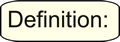Partition means to separate or to divide. A line segment can be partitioned into smaller segments which are compared as ratios.

Partitions occur on line segments that are referred to as directed segments. A directed segment is a segment that has distance (length) and direction. It is important to understand that a directed segment has a "starting point" referred to as the "initial point".

Normally, when working with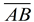it does not matter whether we consider point A first or point B first. But ifis a directed segment, we will be starting at point A (the initial point) and moving in the direction of point B. This direction will be used to clarify the location of the partition ratio on the segment.Partitions and Ratios:
Partitioninginto a 2:3 ratio implies starting at point A. The ratio of 2:3 indicates that the segment will actually be divided into 5 equal sections. If P is the partition point, it will be located 2 of the equal sections away from A and 3 of the equal sections away from B. Consequently, P will be closer to A than it will be to B, since A is the initial point. Notice how, in the examples below, all situations start from point A.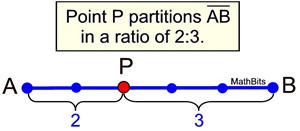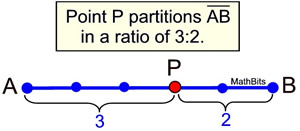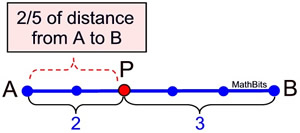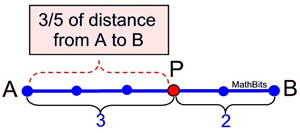Partition Ratio: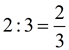compares AP to PB. (the sections of length AB) AP Distance from A Ratio: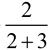=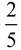compares AP to AB. (a portion of the total length AB)
Did you notice that to get the distance from A to P to be 2/5 of the distance from A to B,
you simply
add the numerator of the partition ratio to its denominator.When working on a coordinates axes, there are several ways to find the coordinates of a "partition" point of a line segment. Let's look at some of the choices.Partitioning using Similar Triangles:
 As shown at the right, if P partitions directed segmentin a ratio of 2:3, find the coordinates of P. Solution: If we draw the yellow and green right triangles as shown, we have two similar right Δs. Each triangle has a right angle and the sharing of angle A which will satisfy the AA Theorem to prove triangles similar. These Δs also indicate the change in vertical distance ("rise") and change in horizontal distance ("run") in each triangle.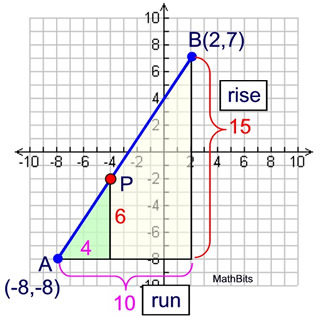In this situation, P is 2/5 of the distance from A to B, giving AP = (2/5) AB. The ratio of similitude between the two similar triangles is 2/5. Since the corresponding sides of similar Δs are in proportion, we know the "rise" associated with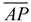will be 2/5 of the "rise" associated with, and the "run" associate withwill be 2/5 of the "run" associated with.
RUN associated with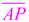: (2/5)(10) =           RISE associate with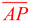: (2/5)(15) = 6

Simply "add" these lengths to the coordinates of A to locate the coordinates of P.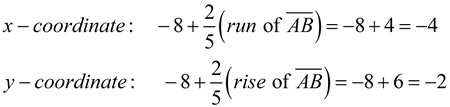P(-4,-2)Partitioning using a Formula:
 The concepts in the method shown above can be expressed as variables to produce a formula. • Let the endpoints be A(x1, y1) and B(x2, y2). • The "run" is the change in the x-coordinates: (x2 - x1) • The "rise" is the change in the y-coordinates: (y2 - y1) • For the partition ratio, let the numerator = a and the denominator = b.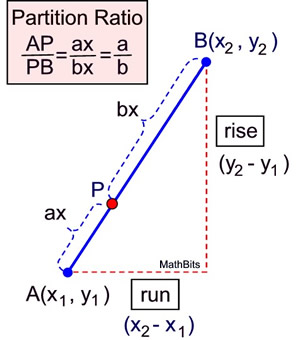The coordinates of P can be found using the formula: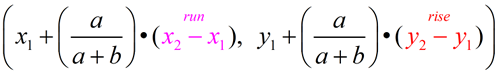While this is a great algebraic formula, it is unlikely that you are going to remember this formula. Instead, simply remember the concept that was presented in the similar triangle explanation.Partitioning using Dilations:
Does the formula we just saw look vaguely familiar? Okay! It may not look familiar, but it does have many of the components of another formula we have seen.

Since we have been dealing with similar triangles, the concept of dilations may come to mind.

Formula for a dilation, center not at the origin: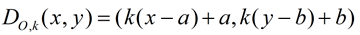O = center of dilation at (a,b);    k = scale factor

Regarding directed line segment, we will be dilating the endpoint B using the endpoint A as the center of the dilation. Since our partition point lies ON the segment, we will be dealing with a dilation which is a reduction (0 < k < 1).
The image of the dilation will be the partition point, P.

The variables a and b do not represent the same quantities in the two formulas we are examining, so if we tweek this dilation formula to correspond to our given information, we may see the resemblence between the formulas more clearly.
Remember, the point being dilated is B, and its image will be the partition point P.
The center of our dilation is point A at (x1, y1), so we will replace a with x1 and b with y1.
The point being dilated is B, so we will replace x with x2 and y with y2.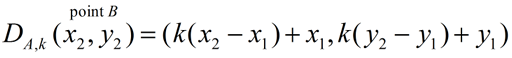If we rearrange a couple of terms (commutative property), we will get: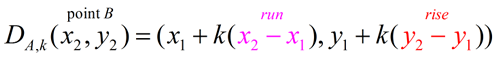Let's compare this improved dilation formula to our previous formula for finding P:Notice: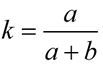The scale factor of the dilation, k, is equal to the ratio of AP to the total length AB.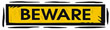If you choose to use this dilation method when partitioning segments, remember that the "partition ratio" (2/3 in this case) is NOT the scale factor. The "AP distance ratio" representing a portion of the whole segment length AB (2/5 in this case) is the scale factor.